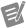# Interpolate 1D Array (G Dataflow)

Calculates a decimal y-value from an array of numbers or points at a specified fractional index or x-value using linear interpolation.## array

An array containing one of the two following data types:

• An array of numbers representing y values equally spaced along the x-axis.
• An array of points where each point is a cluster of x and y coordinates.

If this input is an array of points, the node uses the first element in the cluster (x) to obtain a fractional index by linear interpolation. The node then uses this fractional index to compute the output y value from the second cluster element (y).## fractional index or x

The index or x-value at which the node should return a y-value. fractional index or x must be located directly on a point or between two points for the node to work correctly.

fractional index or x does not interpolate beyond the bounds of an array or data point set. For example, if the parameter is set lower than the first element or x-value in an array, the node returns the value of the first element or the y-value of the first data point. Similarly, if the parameter is set higher than the last element or x-value in the array, the node returns the value in the final element or the final y-value.## y value

The interpolated value of the element at the fractional index or the interpolated y-value of the fractional data point in array.

## Interpolating an Array of Numeric Values

If you wire an array of numeric values, Interpolate 1D Array interprets fractional index or x as a reference to the array elements.

## Interpolating an Array of Data Point Sets

If you wire an array of data point sets, Interpolate 1D Array interprets fractional index or x as a reference to the x-value elements within each data point set.Note

If you wire an array of data points to this node, the data points must be sorted by increasing x-value. If the data points are not sorted by increasing x-value, the node returns invalid results.

array fractional index or x y value comments
[5, 7] 0.5 6.0 The output is halfway between the values at elements 0 and 1.
Array of points

[(3,7), (5,9)]

3.5 7.5 The output is the linearly interpolated value at the x-value corresponding to 3.5.

Where This Node Can Run:

Desktop OS: Windows

FPGA: DAQExpress does not support FPGA devices